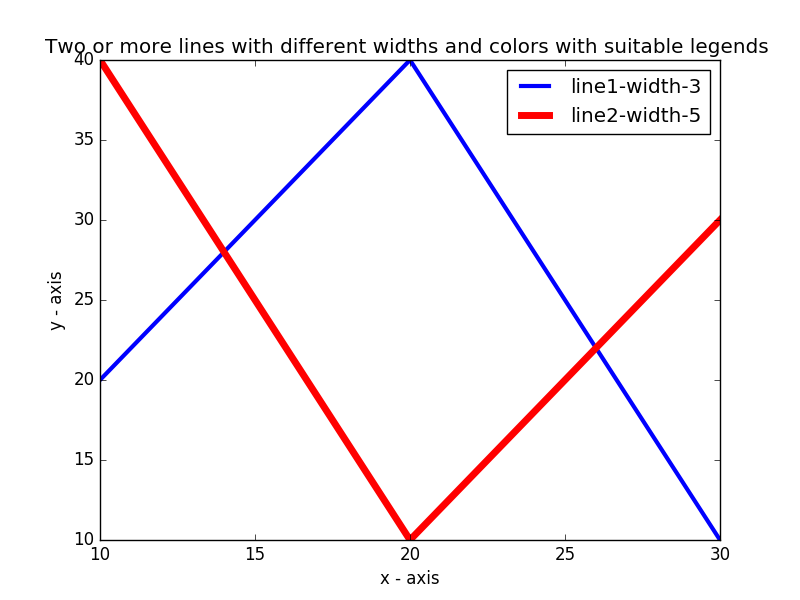﻿ Matplotlib Basic: Plot two or more lines with legends, different widths and colors - w3resource# Matplotlib Basic: Plot two or more lines with legends, different widths and colors

## Matplotlib Basic: Exercise-6 with Solution

Write a Python program to plot two or more lines with legends, different widths and colors.

Sample Solution:

Python Code:

``````import matplotlib.pyplot as plt
# line 1 points
x1 = [10,20,30]
y1 = [20,40,10]
# line 2 points
x2 = [10,20,30]
y2 = [40,10,30]
# Set the x axis label of the current axis.
plt.xlabel('x - axis')
# Set the y axis label of the current axis.
plt.ylabel('y - axis')
# Set a title
plt.title('Two or more lines with different widths and colors with suitable legends ')
# Display the figure.
plt.plot(x1,y1, color='blue', linewidth = 3,  label = 'line1-width-3')
plt.plot(x2,y2, color='red', linewidth = 5,  label = 'line2-width-5')
# show a legend on the plot
plt.legend()
plt.show()
```
```

Sample Output:Python Code Editor: